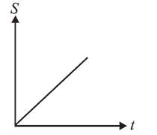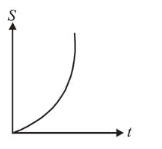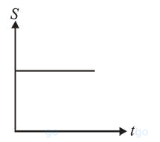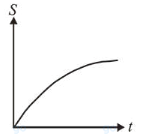# A particle is moving unidirectionally on a horizontal plane under the action of a constant power supplying energy source.`
Question:

A particle is moving unidirectionally on a horizontal plane under the action of a constant power supplying energy source. The displacement $(s)$ - time $(t)$ graph that describes the motion of the particle is (graphs are drawn schematically and are not to scale) :

1.2.3.4.Correct Option: , 2

Solution:

(2) We know that

Power, $P=F v$

But $F=m a v=m \frac{d v}{d t} v$

$\therefore P=m v \frac{d v}{d t} \Rightarrow P d t=m v d v$

Integrating both sides $\int_{0}^{t} P d t=m \int_{0}^{v} v d v$

P. $\mathrm{t}=\frac{1}{2} m v^{2} \Rightarrow v=\left(\sqrt{\frac{2 P}{m}}\right) t^{1 / 2}$

Distance, $s=\int_{0}^{t} v d t=\sqrt{\frac{2 P}{m}} \int_{0}^{t} t^{1 / 2} d t=\sqrt{\frac{2 P}{m}} \cdot \frac{t^{3 / 2}}{3 / 2}$

$\Rightarrow s=\sqrt{\frac{8 P}{9 m}} \cdot t^{3 / 2} \Rightarrow \mathrm{s} \propto \mathrm{t}^{3 / 2}$